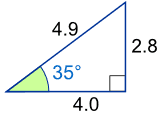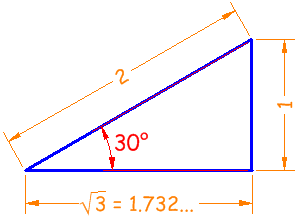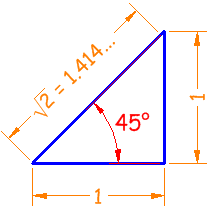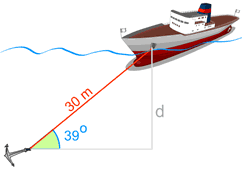# 正弦、余弦和正切

## 直角三角形• "对边" 是在角 θ 的对面
• "邻边" 是在角 θ 的旁边
• "斜边" 是长的一边邻边 是在角的旁边 对边 是在角的对面

## 正弦、余弦和正切### 例子： 35°的正弦是多少？sin(35°) = 对边 / 斜边 = 2.8 / 4.9 = 0.57... cos(35°) = 邻边 / 斜边 = 4.0 / 4.9 = 0.82…… tan(35°) = 对边 / 邻边 = 2.8 / 4.0 = 0.70……## Sohcahtoa

 Soh... Sine = Opposite （对边） / Hypotenuse （斜边） ...cah... Cosine = Adjacent （邻边） / Hypotenuse （斜边） ...toa Tangent = Opposite （对边） / Adjacent （邻边）

## 例子

### 例子： 30° 的正弦、余弦和正切是什么？正弦 sin(30°) = 1 / 2 = 0.5 余弦 cos(30°) = 1.732 / 2 = 0.866... 正切 tan(30°) = 1 / 1.732 = 0.577...

（用计算器来检查答案！）

### 例子： 45° 的正弦、余弦和正切是什么？正弦 sin(45°) = 1 / 1.414 = 0.707... 余弦 cos(45°) = 1 / 1.414 = 0.707... 正切 tan(45°) = 1 / 1 = 1

## 为什么？

• 因为当我们知道边长时，我们可以用它们来计算角度
• 同时，当我们知道角度时，我们也可以用它们来计算边长### 例子： 用 正弦函数 来计算 "d"

• 电缆与海底成 39° 的角
• 电缆长度为 30 米

 这样开始： sin 39° = 对边/斜边 sin 39° = d/30 两边互换： d/30 = sin 39° 用计算器来求 sin 39°: d/30 = 0.6293… 两边乘以 30： d = 0.6293… x 30 d = 18.88 计算结果保留两位小数。

## 不常见的函数

 正割 函数： sec(θ) = 斜边 / 邻边 (=1/cos) 余割 函数： csc(θ) = 斜边 / 对边 (=1/sin) 余切 函数： cot(θ) = 邻边 / 对边 (=1/tan)